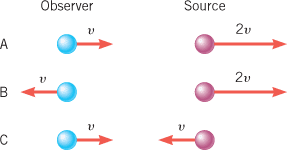# Electromagnetic waves, doppler effect

I pay for chegg to help me with physics but sometimes I'm not quite sure if the site is right. Could you please tell me if I am doing the below problem correctly? My way is different than Chegg's so I would just like to make sure I am not wrong because I am getting different answer than that site :) Thank you!PLEASE DOUBLE CLICK ON THE ABOVE PIC TO SEE FULL DETAILS AND MAGNITUDES! Please double click twice! When you first double click you will not see the magnitudes because it is on a black background. So please double click again to see the magnitudes!

he drawing shows three situations—A, B, and C—in which an observer and a source of electromagnetic waves are moving along the same line. In each case the source emits a wave that has a frequency of . The arrows in each situation denote velocity vectors of the observer and source relative to the ground and have the magnitudes indicated (v or ), where the speed v is . Calculate the observed frequency in each of the three cases.

## Homework Equations

fo = fs(1 + - Vr/C)
Vr = 1.5E6 m/s
fs 4.57E14

## The Attempt at a Solution

a.
here it is relative velocity is just Vr and it is a (-) sign because the faster object is outpacing the slower object and thus they are receding from one another or moving away
fo =(4.57E14)(1 - 1.5E6/3E8)
fo = 4.55E14 hz

b.
here relative velocity is 3Vr and it is a minus sign because the objects are moving away from one another
fo =(4.57E14)(1 - 3*1.5E6/3E8)
fo = 4.501E14 hz

c.

here it is 2Vr and a + sign because the objects are moving towards each other

fo = (4.57E14)(1 + 2*1.5E6/3E8)
fo = 4.62E14 hz

Last edited:

TSny
Homework Helper
Gold Member
fo = fs(1 + - Vr/C)
Vr = 1.5E6 m/s
fs 4.57E14

## The Attempt at a Solution

a.
here it is relative velocity is just Vr and it is a (-) sign because the faster object is outpacing the slower object and thus they are receding from one another or moving away
fo =(4.57E14)(1 - 1.5E6/3E8)
fo = 4.57E14 hz

b.
here relative velocity is 3Vr and it is a minus sign because the objects are moving away from one another
fo =(4.57E14)(1 - 3*1.5E6/3E8)
fo = 4.52E14 hz

For parts a and b it looks to me that you have it set up correctly but you're off a bit in the evaluation. I get different answers for the third digit. I think your answer to part c is correct.

whoops edited. thank you so much!